Yao, J., Ma, L., Lu, W., and Tan, H. (2015). "Tensile property analysis and prediction model building for coir rope reinforced unsaturated polyester composite," BioRes. 10(1), 697-708.

#### Abstract

Because of the light weight and environmental advantages of natural fibers, an increasing amount of natural fibers have been used to replace synthetic fibers in reinforced unsaturated polyester (UPE). Because of the impact property advantage of coir fibers, coir toughened UPE composites can achieve excellent impacting toughness, but at the cost of a lower tensile performance. In order to get the better comprehensive performance, the tensile strength must be maintained in a higher level, so coir ropes as an appropriate reinforced form were added to UPE matrix. The different weight-percent contents for the coir rope addition were set to achieve coir rope reinforced UPE composites with different coir contents. The tensile test results showed increasing tensile strength with the increased content of coir ropes. To reasonably and accurately predict the composite performance, taking the original performance prediction model based on a continuous reinforced fiber composite (using the Classical Mixed Law as a reference) and assuming each coir rope was ideally continuous fiber, the destructive principle of coir rope reinforced UPE composite under the action of tensile load was analyzed and the tensile failure mechanics model was improved. According to the experimental proof, the new model can be proven to have higher precision accuracy, which can provide new train of thought for the building of the theoretical models for natural fiber reinforced composites, thus guiding the actual production application.

#### Full Article

Tensile Property Analysis and Prediction Model Building for Coir Rope Reinforced Unsaturated Polyester Composite

Jia Yao,a,b Lili Ma,a Wei Lu,a,b,* and Haiyan Tan c

Because of the light weight and environmental advantages of natural fibers, an increasing amount of natural fibers have been used to replace synthetic fibers in reinforced unsaturated polyester (UPE). Because of the impact property advantage of coir fibers, coir toughened UPE composites can achieve excellent impacting toughness, but at the cost of a lower tensile performance. In order to get the better comprehensive performance, the tensile strength must be maintained in a higher level, so coir ropes as an appropriate reinforced form were added to UPE matrix. The different weight-percent contents for the coir rope addition were set to achieve coir rope reinforced UPE composites with different coir contents. The tensile test results showed increasing tensile strength with the increased content of coir ropes. To reasonably and accurately predict the composite performance, taking the original performance prediction model based on a continuous reinforced fiber composite (using the Classical Mixed Law as a reference) and assuming each coir rope was ideally continuous fiber, the destructive principle of coir rope reinforced UPE composite under the action of tensile load was analyzed and the tensile failure mechanics model was improved. According to the experimental proof, the new model can be proven to have higher precision accuracy, which can provide new train of thought for the building of the theoretical models for natural fiber reinforced composites, thus guiding the actual production application.

Keywords: Tensile property; Coir rope reinforced UPE; Continuous fiber; Performance predict model

Contact information: a: College of Mechanical Engineering, Jiamusi University, 154007, Jiamusi, China; b: Biomass Comprehensive Utilization Technology of Research Institute, Jiamusi University, 154007, Jiamusi, China; c: College of Material Science and Engineering, Northeast Forestry University, Harbin, 150040, China; *Corresponding author: lujunqi2001@163.com

INTRODUCTION

In recent years, an increasing number of studies have focused on natural fiber reinforced UPE. The main purpose behind adding natural fibers in UPE has been to improve the toughness of the matrix resin, thus overcoming the brittleness defects of UPE. In addition, natural fiber can reduce the cost of the composite as well as accentuate the lightweight design of the composite, when compared to glass fiber and other synthetic fibers.

For natural fiber reinforced UPE (such as with sisal, jute, or flax), comparisons of the performance of glass fiber reinforced UPE has been discussed (Li et al. 2000). The comparative study found that the bending and tensile strength was the highest for jute fiber, but the impact toughness for jute fiber, as well as the mechanical properties and waterproof properties of sisal fiber, were the poorest for all natural fibers.

Non-woven hemp felt reinforced UPE composites and short chopped woven glass fiber reinforced UPE composites were prepared. The study found that the hemp reinforced composite showed a higher degree of toughness than the glass fiber reinforced composite (Hughes et al. 1999). Jains studied bamboo fiber reinforced UPE and found that the strength of the bamboo fiber reinforced UPE composites was better than that of the glass fiber reinforced composite (Jains 1996). O’Dell used non-woven jute fiber during the preparation of the UPE composite plate (O’Dell 1997). The tensile strength and the tensile and bending modulus of the jute sample were half of the glass fiber composite plate. Furthermore, the cantilever beam impact strength and bending strength were also lower than the glass fiber reinforced composite (O’Dell 1997). Mechanical properties, such as the tensile, flexural, and compressive strengths of sisal/silk and coir/silk unsaturated polyester based hybrid composites with different fiber lengths were studied (Khanam et al. 2007; Khanam et al. 2010). Generally, the tensile properties of the composites were improved significantly by adding fibers to a polymer matrix, as the fibers possess much higher strength and stiffness values than the matrix. However, this conclusion is only credible when the fibers are synthetic fibers (such as glass fiber), or when the tensile strength of the natural fibers is much stronger than the matrix material. For example, the impact toughness of the coir reinforced UPE was excellent, but the tensile strength was not comparable to that of the UPE matrix (Yao et al. 2013). The natural fibers were further processed into mats, rovings, yarns, and other textile products. Pre-processed flax fibers (Romhány et al. 2006; Charlet et al. 2010), fiber mats (Oksman 2001), and continuous textile reinforcements (Angelov et al. 2007; Zhang and Miao 2010) have been applied to produce oriented, quasi-unidirectional flax/polymer composites. Furthermore, some mechanical properties of the composites can be improved through the form alterations.

Although the performance prediction theory of fiber reinforced composites has become increasingly developed, the classic “rule of mixtures” is still the foundation for the theory development. Due to the growth characteristics of natural fibers, performance prediction for natural fiber reinforced composites is more difficult. Theoretical predictions for continuous-fiber composites are obtained using the statistical model developed by Curtin (2000), which accounts for fiber strength scatter and local load sharing. Previously determined elementary flax fiber strength distribution (Andersons et al. 2005) and adhesion between fibers and the polymer matrix (Joffe et al. 2005; Andersons and Joffe 2011) were used in the theoretical model.

In this study, coir rope can be supposed to behave as continuous fiber. Also, the tensile strength can be measured easily, yielding results much lower than the UPE matrix. But the tensile strength showed the tendency of increasing with the increase of coir contents, which is not consistent with the rule of mixtures. Through the microstructure research, both the UPE in the inner of gaps in between each fiber in the interior of the fiber rope and the immersed UPE present in the void spaces of the cells of coir were accounted for as the interfacial interaction term in the paper, and the effect must be considered in the correction prediction model.

In summary, the changing principle of tensile strength of the coir rope reinforced UPE were analyzed. Based on this analysis, more reliable tensile properties prediction models were established.

EXPERIMENTAL

Materials

Coir ropes used as the reinforced body were bought from Juxin Coconut Palm Products Co. Ltd, Weifang, Shandong province of China. The average coarseness of each coir rope was 0.3 cm and the average mass was 7.0 g/m. UPE TM-196SP used as the matrix material was bought from Changzhou Huari New Material Co., Ltd of China. Butanox M-50, bethyl ethyl ketone peroxide, and cobalt naphthenate were used as hardener, accelerator, and catalyst, respectively for the UPE matrix.

Composite Preparation

Coir ropes were first pre-impregnated into the UPE matrix for 5 minutes to ensure the full dipping of coir fibers with UPE so that the interfaces of the composite were bonded intimately. 2 wt.% hardener, 1 wt.% accelerator, and 1 wt.% catalyst were added into UPE resin by the weight of each resin. The weight percent contents of coir rope addition were selected as 10 wt.%, 15 wt.%, 20 wt.%, 25 wt.% or 30 wt.%. A hand-lay-up method and semi-open mold were adopted in the preparation process. Plain UPE specimens without coir were also produced in the same condition for the comparison research. A 0.05MPa compression pressure was applied using dead weights on the mold for 1 h, and the composite specimens were removed from the mold and cured at room temperature for 24 hours before testing.

Table 1. Experimental Design and Tensile Properties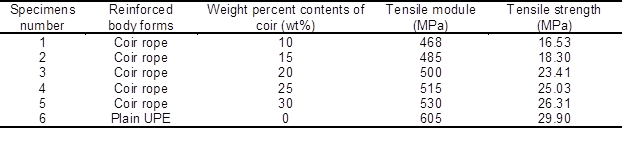Tensile Properties Testing

A RGT-20A microcomputer control electronic universal test machine (made in Shenzhen Ruigeir Instrument Co., LTD of China) was used for the tensile test. The specimens were cut into 180 mm×10 mm×4 mm size for testing. The ISO 527-4:1997 method was adopted as the standard for tensile testing. All tests were carried out at 25 °C temperature and 50% relative humidity. For statistical purposes, the values available were mathematically averaged from five duplicate specimens.

THEORY EQUATION

Mixed Law and Halpin-Tsai Equation

The performance of the continuous fiber reinforced composite can be accurately predicted by the law of mixtures and the Halpin-Tsai Equation (Hull and Clyne 1996). The modulus prediction equation is shown as Eq. 1, and the assumptions of Eq. 1 were that there was enough fiber length and the same strain was used for both the fiber and matrix. The axial modulus prediction equation was,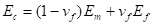(1)

where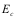is the axial elastic modulus of the composite (MPa);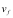is the volume fraction of the fiber;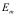is the axial elastic modulus of the matrix (MPa); and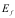is the axial elastic modulus of the fiber (MPa).

Under the same assumptions and conditions, the rule of mixtures can also realize the strength performance prediction of the composite, merely by replacing the elastic modulus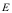with strength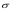, as shown below in Eq. 2.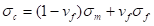(2)

where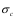is the axial strength of the composite (MPa);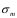is the axial strength of the matrix (MPa);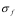is the axial strength of the fiber (MPa).

Tensile Strength Analysis of Continuous Fiber Reinforced Composite

For continuous synthetic fiber reinforced composites, the consistency of both the fiber length and matrix length can be ensured, as shown in Fig. 1. When the tensile force was parallel to the fiber direction of the composite, its tensile strength followed the rule of mixtures (Eq. 2). The axial stress strain curve of fiber reinforced composites can be divided into three phases, as shown in Fig. 2 (Zhao et al. 2006).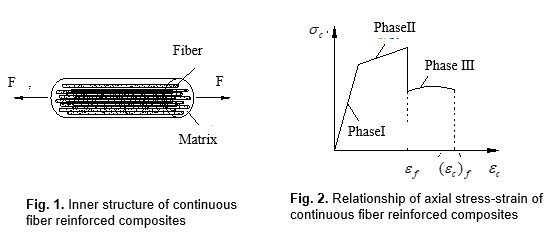In Phase I, the strain was small, and both the fibers and matrix experienced elastic deformation,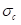, which can be described using Eq. 3.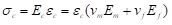(3)

In Eq. 3,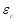represents the axial elastic strain of the composite.

The tensile strength of the fiber was high under this condition, as the fibers were still in the stage of elastic deformation that takes place in Phase II; however, the matrix experienced plastic deformation,, which can be further described in Eq. 4,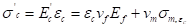(4)

where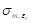is the stress of the matrix when the strain is(MPa), which can be determined by the tensile test of the matrix material.

When the matrix part was broken under the tensile load, the composite strength suddenly fell, and the composite deformation entered Phase III. During this phase, the fiber parts endured plastic deformation, and after finally arriving at the critical tensile strength, the fibers were under the critical maximum tensile stress. After achieving the ultimate strain, the fiber part fractured in accord with composite as a whole.

The aforementioned theory can predict the properties of continuous fiber reinforced composites. Although the theory does not provide general predictions for natural fiber reinforced composites, it can provide a reference for the analysis that follows.

Results and analysis

Tensile Properties Analysis of Coir Rope Reinforced UPE

The internal structure of coir rope is shown in Fig. 3. A number of coir fibers are curled up along the same circular spiral curl, forming the share of fiber rope that is shown in Fig 3 (a), where the rotational angles of coir were almost the same. During the spindle winding process, the exact amount of coir fibers needed to be constantly added to keep the length of the coir rope consistent. For the coir rope weaving structure (which was equal to the coarseness diameter of the coir fiber), approximately 30 to 35 coir fibers were arranged on each cross section of the rope for every 0.3 cm coarseness of coir rope. For the length of the coir fiber (which was equal to lengthening of the coir fiber along the longitudinal direction), one can assume the coir rope to be continuous fiber. In Fig. 3 (b), the coir rope as a whole showed a long straight appearance, wherein the internal coir fibers in the visible cross section exhibited an oriented characteristic. For coir rope reinforced UPE matrix, the coir ropes were assumed to be continuous fibers for the purpose of the analysis.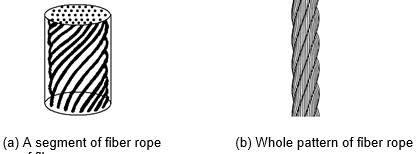Fig. 3. Structure of fiber rope

The tensile fracture of the coir rope reinforced UPE specimen also experienced three phases, as shown in Fig. 4. In Fig. 2, the maximum stress occurred at the end point of Phase II, due to the mechanical performance of the fiber prior to the matrix. By contrast, in Fig. 4 the strength of the coir rope was much smaller than the UPE matrix, and the elongation at the break of the matrix was small, so the maximum stress occurred at the end point of Phase I. In Phase I, the tensile load was shared by both the coir rope and UPE, the strain was small, and both the fibers and matrix endured elastic deformation. At the end of this process, the matrix strain reached its limit, and the ultimate tensile strength of the composite can be obtained. In Phase II, only the coir rope portion endured the tensile load, so its bearing capacity of the maximum load was less than Phase I. The spiral structure of the coir rope was destroyed gradually as the loading increased. The directional property of the coir fibers changed along the direction of the tensile load, which presented a better uniform direction throughout the damaged section. According to the theory that the axial strength of fiber along the load direction was the highest, the bearing capacity of the coir rope portion will change in accordance with the change in the fiber direction. Therefore, the tensile force appeared to exhibit a rising trend in Phase II. After reaching the tensile limit for the portion of coir rope, the structure of the coir rope was completely destroyed and the composite entered Phase III. During Phase III, a tensile load was borne by each composition fiber of coir rope, and a gradual destruction based on fiber morphology and different carrying capacity occurred. Therefore, the bearing capacity of the total composite decreased gradually before the composite fractured completely.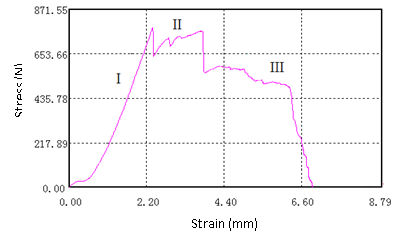Fig. 4. Tensile stress and strain curve of coir fiber rope reinforced UPE

In summary, the fiber rope portion, taken as a whole, will endure tensile load in Phase I and Phase II; however, during Phase III, the overall structure of the coir rope was destroyed, as the composite withstood the tensile load by relying on coir fibers in the orientation distribution form. This change was determined by the difference between the structure of the coir rope and continuous fiber reinforcement.

Tensile Modulus Prediction of Coir Rope Reinforced UPE

As can be seen in Fig 4, when the displacement of the specimen reached approximately 2.5 mm (corresponding to the maximal peak value of the tensile force for the specimen), which corresponds with the end point of Phase I (small strain, elastic deformation of fibers and matrix in Fig. 2), the UPE portion reached its tensile elongation limit and suffered damage. Thus, the tensile modulus can be configured as Eq. 5,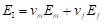(5)

where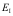is the axial tensile modulus of composite in Phase I, (MPa);is the axial tensile modulus of the coir rope, (MPa);is the axial tensile modulus for the UPE matrix, (MPa);is the volume fraction of the coir rope part; and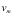is the volume fraction of the UPE portion.

The transformation law of quality percentage and volume percentage can be deduced by the Kelly-Tyson Modified Rule of Mixtures: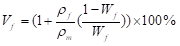(6)

where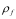is the density of the fiber, (g/cm3);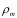is the density of the matrix, (g/cm3); and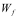is the weight percentage (%).

Table 2. Prediction Values and Measured Values of the Tensile Module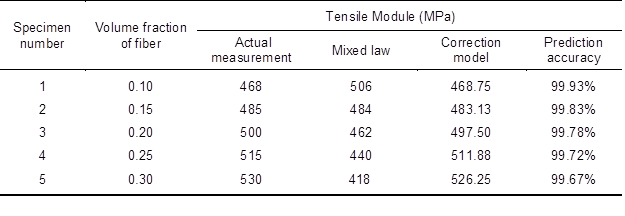According to the experimental results of ISO2811, the UPE density is 1.15 g/cm3 . The coir density is 1.15 g/cm3. Substituting the aforementioned figures into Eq. 6, the consistency of the values for the weight percentage and volume percentage of coir fiber can be obtained, and are shown in Table 2. According to GB/T2568-1995, the measured values for the tensile elastic modulus of the UPE casting body and coir rope were 550 and 110 MPa, respectively. The performance test results for the different contents of coir rope reinforced UPE, as well as the prediction values, can be obtained by substituting the aforementioned data into Eqs. 5 and 6, in accordance with the rule of mixtures. The results are given in Table 2.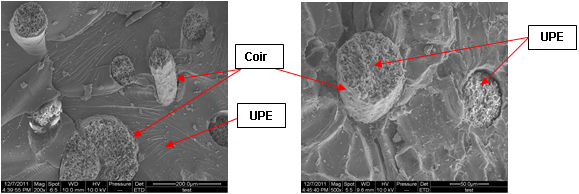(a) total tensile facture                                     (b) UPE in the coir cell wall

Fig. 5. SEM of coir rope reinforced UPE under tensile load

As a result, the tensile elastic modulus of coir rope portion was less than that of the UPE portion; the predictive values of the Mixed Law should gradually be reduced, corresponding to the increase in the coir addition, as shown in Table 2. However, in the coir rope volume range of 10% to 30%, the actual measurement values were greater than the predicted values. This inconsistence can be explained by the structural characteristics of the coir rope portion, because of the existence of gaps in each fiber in the interior of the fiber rope; the unsaturated polyester was immersed in the inner of the rope as in Fig. 5(a) and (b), which allowed the expansion of the interface area of the UPE and coir. From Fig. 5(b), it can be found that the UPE saturated in the cell wall of coir, the UPE inner the coir can play a reinforcing role under the tensile load. The tensile modulus of both the UPE in the inner of gaps in between each fiber in the interior of the fiber rope and the immersed UPE inner the coir was higher than the coir rope part, so the increasing tendency of the tensile performance can be explained better.

In regards to correcting the rule of mixtures equation (Eq. 2), the influence of interfacial interactions, including both the UPE in the inner of gaps in between each fiber in the interior of the fiber rope and the UPE immersed in coir cell wall, should be considered. Therefore, besides the matrix and fiber items in the rule of mixtures equation, the interface interaction item, which strength was supposed higher thanbut lower than, expressing as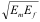, was added in the prediction model. Adding the corresponding adjustment coefficient (less than 1) to the matrix item and the fiber item of coir and UPE, the volume fraction of the interface can be written as: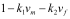. In order to reflect the upward trend of the test values in Table 2, the influence of coir rope adding can be expressed as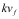(which must be more than 1) in the interface interaction item. According to the measured test data, using Matlab data processing software to program and process the data fitting, the traditional rule of mixtures can be rewritten as follows,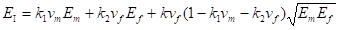(7)

where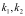are the influence coefficients of the matrix and fiber on the tensile strength respectively (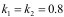in this study); and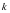is the adjustment coefficient (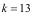in this study).

Substituting the original data into Eq. 7, the correction values obtained using the new model are shown in Table 2. The data precision value can be acquired using to the correction equation, and Eq. 7 can been demonstrated to better predict the trend of the tensile modulus within the fiber volume content scope of 10% ~ 30%. There were smaller deviations with measured values, as the prediction accuracy was higher than 99%.

Tensile Strength Prediction of Coir Rope Reinforced UPE

The measured value for the tensile strength of the UPE casting body was 29.90 MPa, and the actual measured tensile strength of coir rope was 10.10 MPa. The tensile strength of the rule of mixtures for the composite is shown in Table 3. Therefore, in correcting Eq. 4 of the traditional rule of mixtures, according to the method above, the tensile strength prediction model of coir rope reinforced UPE composite can be established as follows: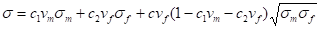(8)

where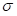is the tensile strength of composite (MPa);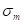is the tensile strength of UPE (MPa);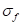is the tensile strength of coir rope (MPa);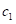and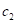are the influence coefficients of the matrix and fiber in terms of tensile strength (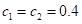in the study); and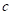is the adjustment coefficient (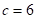in this study).

Table 3. Predicted Values and Measured Values of Tensile Strength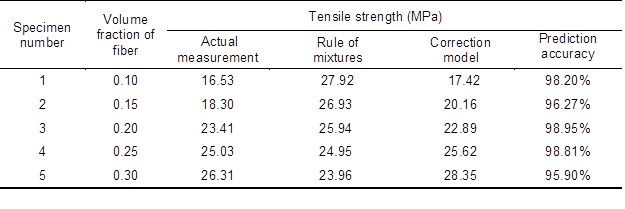Equation 8 has been shown to better predict the trend of the tensile strength of a sample within the fiber volume content scope of 10% to 30%. Finally, there were small deviations with measured values, but the prediction accuracy was still higher than 95%.

Experimental Proof

According to the aforementioned experimental conditions, specimens with weight percentages of 17% and 28% were prepared, and the actual testing of tensile properties was conducted. The tensile module values and the tensile strength values are shown in Table 4.

Substituting the predicted data into Eqs. 7 and 8, respectively, the prediction results and prediction accuracy are shown in Table 4, where the prediction accuracy of the tensile modulus was above 99% and the prediction precision for the tensile strength was above 94%. Therefore, the prediction models for both the tensile module and strength were more reasonable and can effectively predict the tensile performance of coir rope reinforced UPE in the special coir adding ranges of 10% to 30%.

Table 4. Forecast Values and Measured Values of Tensile Strength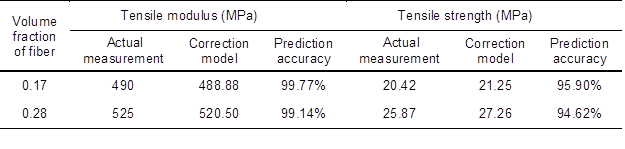CONCLUSIONS

1. Based on the preparation and the performance evaluation of coir rope reinforced UPE composites, the tensile properties of coir rope reinforced UPE composites were obtained. Within the particular scope of coir rope contents (10 wt.% to 30 wt.%), the tensile strength increased by increasing the amount of coir fiber, but it didn’t exceed the tensile strength value of the plain UPE specimen. This phenomenon can be attributed to the strengthening effect of the coir rope form on the matrix affecting the interface between UPE and coir fibers, and the performance reinforcement resulted from the unsaturated polyester penetrating into the internal voids of the coir fiber. So through the optimized design of coir fiber reinforcement form as coir ropes, the tensile performance can keep in a higher level, and the discussed excellent impact performance (Yao et al. 2013) can also be obtained. Thus, coir ropes reinforced UPE composite can be used in place of the traditional UPE material while achieving higher impact performance requirements.

2. The traditional rule of mixtures no longer applied accurately to coir rope UPE composites. Using the tensile fracture theory of continuous synthesis fiber reinforced UPE as a reference, the fracture failure principle of UPE reinforced coir rope under the action of tensile load was analyzed. Assuming the coir ropes as continuous fibers, and after correcting the Mixed Law model by adding an interfacial term and suitable coefficients in the equation, the most suitable tensile performance prediction model for coir rope reinforced UPE was obtained within a particular range of coir rope contents (10 wt.% to 30 wt.%). The predicted results of both the tensile modulus and tensile strength had good prediction accuracy. This study can guide composite design, according to the application requirements, as well as realize the optimization control of additional fiber proportions.

ACKNOWLEDGMENTS

This project was supported by the National Natural Science Foundation of China (51203061), the Heilongjiang Educational Office Scientific Research Program (12541794), and the Youth Backbone Support Plan for Heilongjiang Province Universities (1254G059).

REFERENCES CITED

Andersons, J., Joffe, R., and Wallström L. (2005). “Strength distribution of elementary flax fibres,” Compos. Sci. Technol. 65(3–4), 693-702. DOI: 10.1016/j.compscitech.2004.10.001

Andersons, J., and Joffe, R. (2011). “Estimation of the tensile strength of an oriented flax fiber-reinforced polymer composite,” Composites: Part A 42(9), 1229-1235. DOI: 10.1016/j.compositesa.2011.05.005

Angelov, I., Wiedmer, S., Evstatiev, M., Friedrich, K., and Mennig, G. (2007). “Pultrusion of a flax/polypropylene yarn,” Composites: Part A (5), 1431-1438. DOI: 10.1016/j.compositesa.2006.01.024.

Charlet, K., Jernot, J. P., Gomina, M., Bizet, L., and Bréard, J. (2010). “Mechanical properties of flax fibres and of the derived unidirectional composites,” J. Compos. Mater. 44(24), 2887-2896.  DOI: 10.1177/0021998310369579

Curtin, W. A. (2000). “Tensile strength of fiber-reinforced composites: III. Beyond the traditional Weibull model for fiber strengths,” J .Compos. Mater. 34(15), 1301-1332. DOI: 10.1177/002199830003401503

Hughes, M., Mott, L., and Hague, J. (1999). “The toughness of vegetable fibre-reinforced unsaturated polyester composites,” Fifth International Conference on Woodfiber-Plastic Composites, pp. 175-184.

Hull, D., and Clyne, T. W. (1996). An Introduction to Composite Materials, 2nd ed., Cambridge University Press, Cambridge, UK.

Jani, S., and Kumar, R. (1996). “Mechanical behaviour of bamboo fibres reinforced plastic (BFRP) composite and effect of environment on properties,” Processing and Fabrication of Advanced Materials V, 443-456.

Joffe, R., Andersons, J., and Wallström, L. (2005). “Interfacial shear strength of flax fiber/thermoset polymers estimated by fiber fragmentation tests,” J. Mater. Sci. 40(9-10), 2721-2722. DOI:10.1007/s10853-005-2115-4

Khanam, P. N., Reddy. M. M., Raghu, K., John, K., and Naidu, S. V. (2007). “Tensile, flexural and compressive properties of sisal/silk hybrid composites,” Journal of Reinforced Plastics and Composites 26(10), 1065-1070. DOI:10.1177/0731684407079347

Khanam, P. N., Reddy, G. R., Raghu, K., and Naidu, S. V. (2010). “Tensile, flexural, and compressive properties of coir/silk fiber-reinforced hybrid composites,” Journal of Reinforced Plastics and Composites 29(14), 2124-2127. DOI: 10.1177/0731684409345413

Li, Y., Mai, Y. W., and Ye, L. (2000). “Sisal fibre and its composites: A review of recent developments,” Compos. Sci. Technol. 60 (11), 2037-2055. DOI: 10.1016/S0266-3538(00)00101-9

O’Dell, J. L. (1997). “Natural fibers in resin transfer molded composites,” Fourth International Conference on Woodfiber-Plastic Composites, pp. 280-285.

Oksman, K. (2001). “High quality flax fibre composites manufactured by the resin transfer moulding process,” J. Reinf. Plast. Compos. 20(7), 621-627. DOI: 10.1177/073168401772678634

Romhány, G., Czigány, T., and Karger-Kocsis, J. (2006). “Determination of J–R curves of thermoplastic starch composites containing crossed quasi-unidirectional flax fiber reinforcement,”Compos. Sci. Technol. 66(16), 3179-3187. DOI: 10.1016/j.compscitech.2005.01.016

Yao, J., Lu, W., Rong, S. F., and Hu, Y. C. (2013). “Mechanical properties evaluation of coir toughened unsaturated polyester with different reinforced body forms,” BioResources 8(3), 3336-3346.

Zhang, L., and Miao, M. (2010). “Commingled natural fibre/polypropylene wrap spun yarns for structured thermoplastic composites,” Compos. Sci. Technol. 70(1), 130-135. DOI: 10.1016/j.compscitech.2009.09.016

Zhao, X. B., Ling, G. P., and Qian, G. D. (2010). The Performance of the Materials, Higher Education Press, Beijing, China.

Article submitted: June 28, 2014; Peer review completed: September 1, 2014; Revised version received and accepted: November 18, 2014; Published: December 3, 2014.